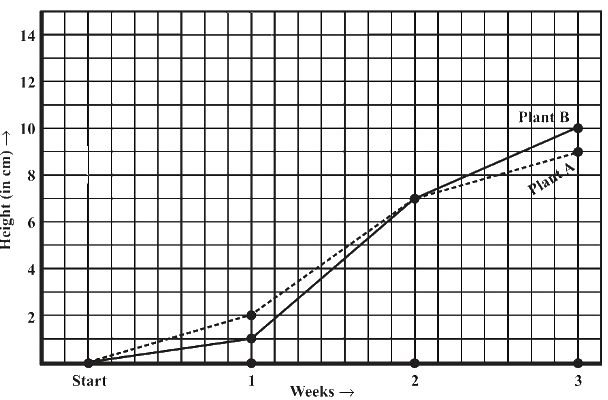# Ex.15.1 Q3 Introduction to Graphs Solutions - NCERT Maths Class 8

Go back to  'Ex.15.1'

## Question

For an experiment in Botany, two different plants, plant $$A$$ and plant $$B$$ were grown under similar laboratory conditions. Their heights were measured at the end of each week for $$3$$ weeks. The results are shown by the following graph.

(a) How high was Plant $$A$$ after (i) $$2$$ weeks (ii) $$3$$ weeks?

(b) How high was Plant $$B$$ after (i) $$2$$ weeks (ii) $$3$$ weeks?

(c) How much did Plant $$A$$ grow during the $$3^\rm{rd}$$ week?

(d) How much did Plant $$B$$ grow from the end of the $$2^\rm{nd}$$ week to the end of the $$3^\rm{rd}$$ week?

(e) During which week did Plant $$A$$ grow most?

(f) During which week did Plant $$B$$ grow least?

(g) Were the two plants of the same height during any week shown here? Specify.Video Solution
Introduction To Graphs
Ex 15.1 | Question 3

## Text Solution

Reasoning:

Horizontal axis represents ‘Weeks’ and vertical represents ‘Height’ in $$\rm{cm.}$$

We can find the value of ‘Height’ on vertical axis according to the given value of ‘Weeks’ on the horizontal axis value or vice versa.

Steps:

(a) How high was Plant $$A$$ after (i) $$2$$ weeks (ii) $$3$$ weeks?

(i) After $$2$$ weeks, the height of plant $$A$$ was $$7 \;\rm{cm.}$$

(ii) After $$3$$ weeks, the height of plant A was $$9 \;\rm{cm.}$$

(b) How high was Plant $$B$$ after (i) $$2$$ weeks (ii) $$3$$ weeks?

(i) After $$2$$ weeks, the height of plant $$B$$ was $$7 \;\rm{cm.}$$

(ii) After $$3$$ weeks, the height of plant $$B$$ was $$10 \;\rm{cm.}$$

(c) Growth of plant $$A$$ during $$3$$rd week $$= 9\;\rm{cm} − 7\;\rm{cm} = 2\;\rm{cm}$$

(d) Growth of plant $$B$$ from the end of the $$2^\rm{nd}$$ week to the end of the $$3 ^\rm{rd}$$ week

$$= 10\, \rm{cm} − 7 \, \rm{cm} = 3 \, \rm{cm}$$

(e) Growth of plant $$A$$ during $$1^\rm{st}$$ week $$= 2\,\rm{cm } − 0 \,\rm{cm } = 2 \,\rm{cm }$$

Growth of plant $$A$$ during $$2^\rm{nd}$$week $$= 7 \,\rm{cm} − 2 \,\rm{cm} = 5 \,\rm{cm}$$

Growth of plant A during $$3^\rm{rd}$$ week $$= 9\,\rm{cm} − 7 \,\rm{cm} = 2 \,\rm{cm}$$

Therefore, plant $$A$$ grew the most, i.e. $$5 \,\rm{cm,}$$ during the $$2^\rm{nd}$$ week.

(f) Growth of plant $$B$$ during $$1^\rm{st}$$ week $$= 1\,\rm{cm} − 0 \,\rm{cm} = 1 \,\rm{cm}$$

Growth of plant $$B$$ during $$2^\rm{nd}$$ week $$= 7\,\rm{cm} − 1\,\rm{cm} = 6 \,\rm{cm}$$

Growth of plant $$B$$ during $$3^\rm{rd}$$ week $$= 10 \,\rm{cm} − 7\,\rm{cm} = 3\,\rm{cm}$$

Therefore, plant $$B$$ grew the least, i.e. $$1 \;\rm{cm,}$$ during the $$1^\rm{st}$$ week.

(g) At the end of the $$2^\rm{nd}$$ week, the heights of both plants were same .i.e. $$7 \rm{cm}$$.

Learn from the best math teachers and top your exams

• Live one on one classroom and doubt clearing
• Practice worksheets in and after class for conceptual clarity
• Personalized curriculum to keep up with school